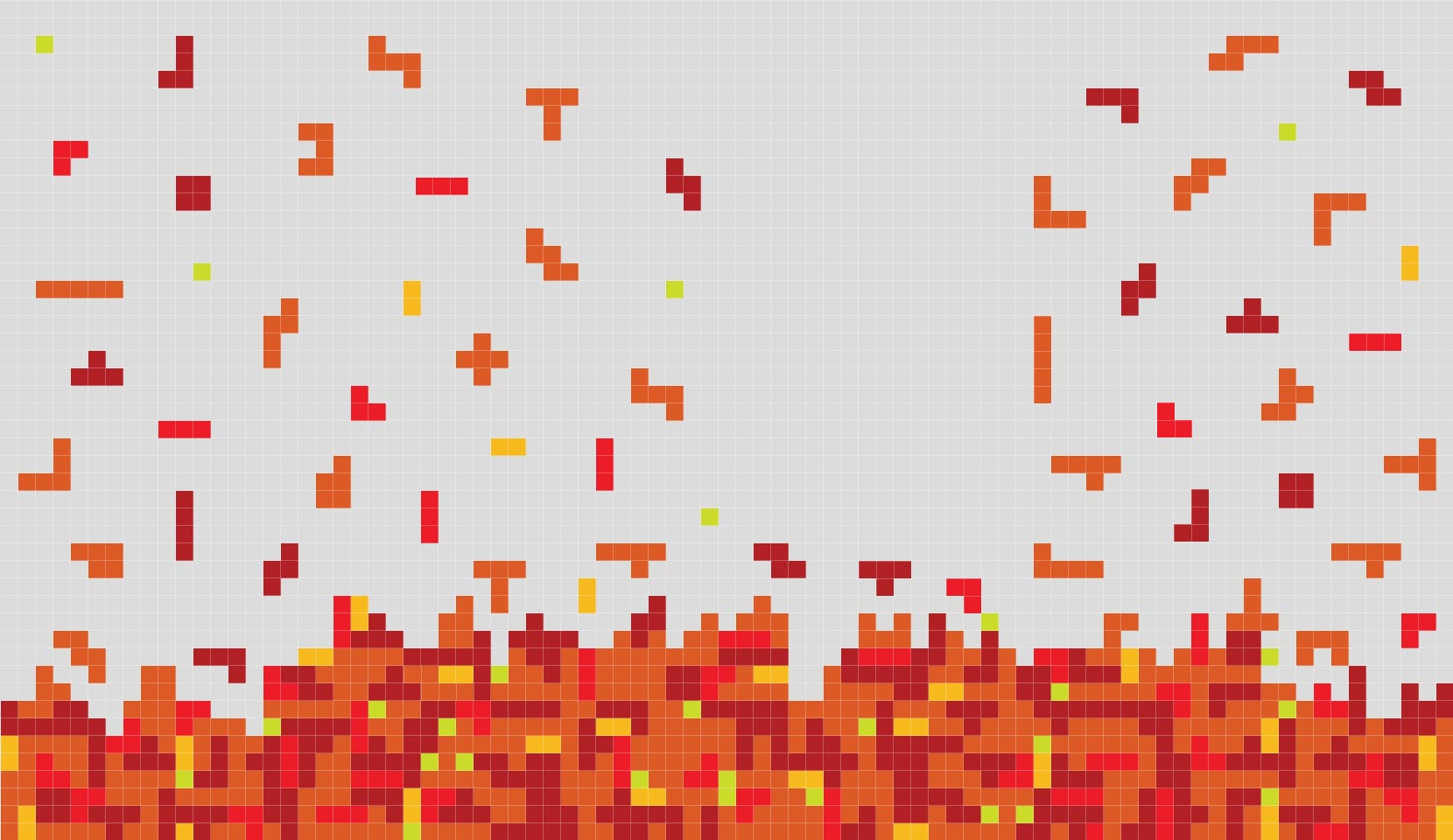Software

Tools for the Investigation of Non-Classical logics

The TINC system, which includes:

AxiomCalc

The system AxiomCalc takes as input an Hilbert axiom specified in the language of Full Lambek Calculus with exchange (i.e. intuitionistic logic without weakening and contraction) and transforms it, if possible, into an equivalent analytic sequent or hypersequent rule.

AxiomCalc

• (i) identifies the class of the syntactic classification in [CGT08] to which the axiom belongs
• (ii) transforms axioms within the class P3 of the classification into equivalent analytic (hyper)sequent rules,
• (iii) generates a paper containing the resulting analytic calculus, and,
• (iv) if explicitly required by the user, checks whether Monoidal T-norm Logic MTL extended with the input axiom is standard complete (i.e. it has a semantics in the real interval [0,1]).
Link to the AxiomCalc software

Paralyzer

Paralyzer (PARAconsistent logics AnaLYZER) tranforms Hilbert calculi (of a certain general form) into analytic sequent calculi, and generates a (nondeterministic) finite-valued semantics for them. The method applies to a variety of well-known logics, including a large family of paraconsistent logics (and that is where the tool takes its name), as well as to other logics for which neither analytic calculi nor suitable semantics have so far been available.

The calculi of Paralyzer are obtained

• (i) by extending the language of the positive fragment of classical logic, to a language which includes also a finite set of new unary connectives, and
• (ii) by adding to a Hilbert axiomatization of classical logic, axioms over the new language of a certain general form.
Link to the Paralyzer software

Framinator

The system Framinator (FRAMe condItioNs Automatically TO Rules) takes as input a frame condition in the language of first-order classical logic provided by the user and transforms it - if possible - into an equivalent labelled rule.
Framinator

• (i) identifies the class of the arithmetical hierarchy to which the frame condition belongs,
• (ii) transforms frame conditions within the class Pi(2) of the arithmetical hierarchy into equivalent structural labelled ruler, and
• (iii)provides a paper containing a cut-free calculus for the logic defined by imposing the frame condition on the standard intuitionistic frame.
Link to the Framinator software